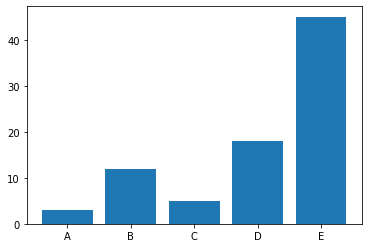This example shows probably the most basic barplot you can do with python and matplotlib. The `bar()` function used with the following parameters:

• `x` : The x coordinates of the bars. (x_pos in the example)
• `height` : The height(s) of the bars.
``````# Libraries
import numpy as np
import matplotlib.pyplot as plt

# Create dataset
height = [3, 12, 5, 18, 45]
bars = ('A', 'B', 'C', 'D', 'E')
x_pos = np.arange(len(bars))

# Create bars
plt.bar(x_pos, height)

# Create names on the x-axis
plt.xticks(x_pos, bars)

# Show graphic
plt.show()``````## Contact & Edit

👋 This document is a work by Yan Holtz. You can contribute on github, send me a feedback on twitter or subscribe to the newsletter to know when new examples are published! 🔥